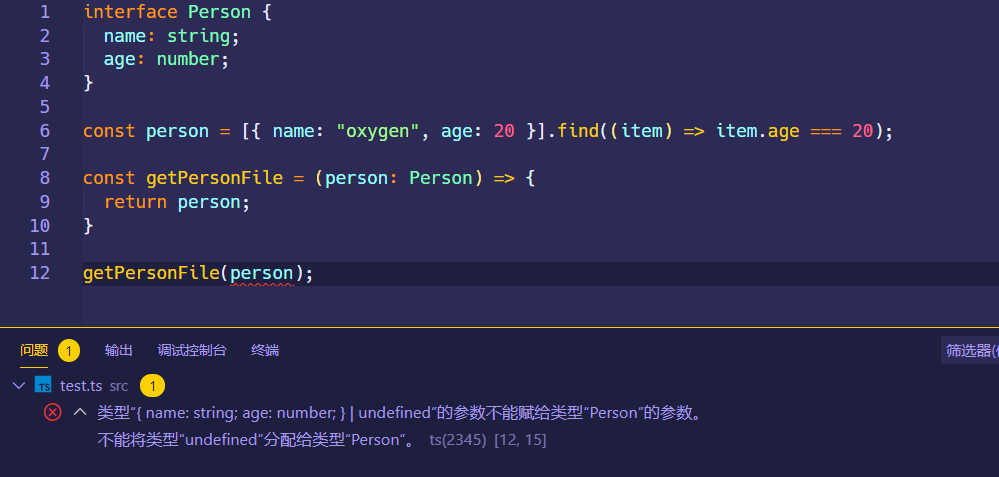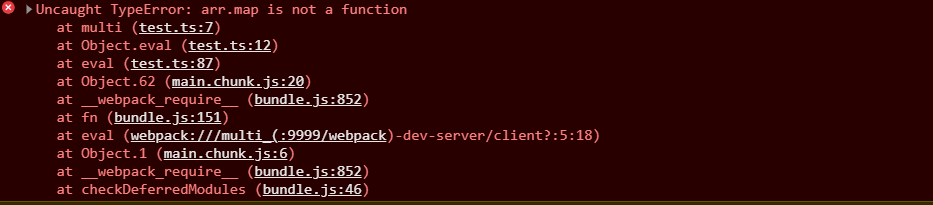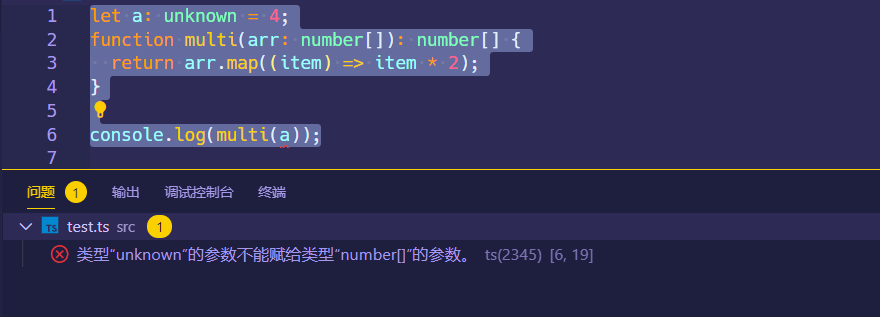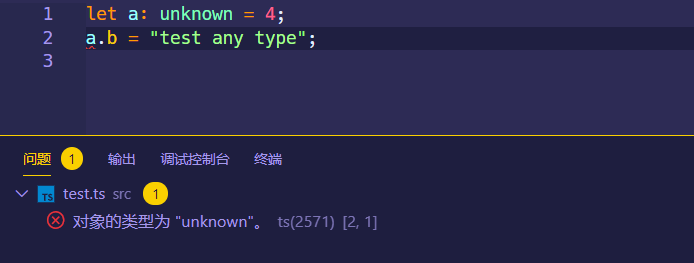# TypeScript类型系统

## ES 原始值类型​

### 原始值类型的表示​

// Booleanlet variable: boolean = false;// Numberlet variable: number = 6;// BigInt, BigInt类型的变量值后面都会带有 nlet variable: bigint = 100n;// Stringlet variable: string = 'str';// Symbollet sym: symbol = Symbol();// Null / Undefinedlet u: undefined = undefined;let n: null = null;

### Null 和 Undefined​

let a: number;a = undefined;

interface Person {  name: string;  age: number;}const person = [{ name: 'oxygen', age: 20 }].find(item => item.age === 20);const getPersonFile = (person: Person) => {  return person;};getPersonFile(person); // error// 方法一const person = [{ name: 'oxygen', age: 20 }].find(item => item.age === 20) || {  name: '',  age: 0,};// 方法二const getPersonFile = (person?: Person) => {  return person;};

// 声明兼容null和undefined的类型let variable: string | null | undefined = 'str';

### 字面量类型​

// string的字面量类型function createElement(tagName: 'input' | 'img'): void {}function handler(eventName: 'click' | 'focus'): void {}// number的字面量类型function add(value: 1 | 2 | 3 | 4 | 5): number {  return value++;}// boolean的字面量类型，直接使用true/false定义interface ValidationSuccess {  isValid: true;  reason: null;}interface ValidationFailure {  isValid: false;  reason: string;}

## ES 引用类型​

### Array​

let list: number[] = [1, 2, 3];

let list: Array<number> = [1, 2, 3];

let list: ReadonlyArray<number> = [1, 2, 3];list.push(4);### Date​

let currentTime: Date = new Date();

### RegExp​

let reg: RegExp = /abc/;

### 函数​

function func(name: string, age: number): string {  return I am ${name},${age} years old.;}

const func: (name: string, age: number) => string = function(  name: string,  age: number,): string {  return I am ${name},${age} years old.;};// 简写const func = function(name: string, age: number): string {  return I am ${name},${age} years old.;};// 或者简写const func: (name: string, age: number) => string = function(name, age) {  return I am ${name},${age} years old.;};// 或者用箭头函数const func: (name: string, age: number) => string = (name, age) => {  return I am ${name},${age} years old.;};

interface Person {  age: number;  getName: (name: string) => string; //定义对象内部的方法}const person: Person = {  age: 20,  getName: name => {    return name;  },};person.getName('oxygen');

interface Func {  (name: string, age: number): string;}// 这里声明的函数的参数名和上面interface内指定的参数名并不需要相同const func: Func = (name, age) => {  return I am ${name},${age} years old.;};func('oxygen', 23);

#### 函数重载​

• 多个重载函数之间只能使用普通函数形式去定义
• 重载只能通过指定不同的参数类型或者参数个数来达到目的，返回类型不属于重载的范围
• 最后一个定义的函数不属于重载函数，其内部需要根据参数的类型进行判断，然后决定执行什么样的操作
function func(name: string): void;function func(birth: Date): void;function func(x: any): any {  if (typeof x === 'string') {    console.log(x);  }  if (typeof x === 'object') {    console.dir(x);  }}func(new Date()); // Mon Oct 05 2020 20:00:01 GMT+0800 (中国标准时间)

### Object​

#### object​

object关键字在 TypeScript 中代表任何非原始值类型，例如一个对象，但是对象表示通常使用interfaceinterface可以准确地表示对象内所包含的属性，以及每个属性的类型，所以object用的很少

let obj: object = { prop: 0 };

## TS 特定类型​

### Enum​

enum Color {  Red,  Green,  Blue,}enum Status {  Init,  Completed,  Success,  Failed,}enum Days {  Sun,  Mon,  Tue,  Wed,  Thu,  Fri,  Sat,}

// 指定枚举项从 1 开始enum Color {  Red = 1,  Green,  Blue,}// 单独指定每个枚举项的值enum Color {  Red = 1,  Green = 2,  Blue = 4,}

export enum EvidenceTypeEnum {  Init = 'init',  Procesing = 'procesing',  Success = 'success',  Failed = 'failed',}

### Any​

let variable = 4;let num: any = 4;

let num: any = 4;num = 'str';

let a: any = 4;function multi(arr: number[]): number[] {  return arr.map(item => item * 2);}console.log(multi(a));### Unknown​

unknown也就是未知类型的意思，unknown是所有其他类型的子类型，所以其他任何类型的变量都可以分配给unknown类型。

let a: unknown = 4;a = 'test unknown type';console.log(a); // test unknown type

let a: unknown = 4;function multi(arr: number[]): number[] {  return arr.map(item => item * 2);}console.log(multi(a));let a: any = 4;a.b = 'test any type';### Void​

void就是空类型，没有类型的意思，通常用于没有返回值的函数声明其返回值的类型

function warnUser(): void {  console.log('This is my warning message');}

### Never​

never表示永远不会出现的类型，通常用于直接抛出异常的函数，或者永远没有返回值的函数

function error(message: string): never {  throw new Error(message);}

nevervoid的区别是never可以分配给其他类型，但是void只能分配给anyvoid。还有一点是指定为never的类型往往只用于内部抛出异常来处理全局错误，但是指定为void的函数比较灵活，可以将它们用在各个地方。

### Tuple​

Tuple，元组，元组类型用于声明元素数量和类型都明确的数组，但是每种元素的类型不必相同，元组在声明的时候必须按顺序指定数组中每个元素的类型。

let x: [string, number] = ['hello', 10];

## 类型断言​

• 最新的as关键字语法：
let someValue: unknown = 'this is a string';let strLength: number = (someValue as string).length;
• 旧式的尖括号语法，不推荐在 React 中使用，因为和 JSX 的语法容易混淆，在@babel/preset-typescript中可以通过设置isTSX配置项选择是否将尖括号语法看作 JSX 进行编译
let someValue: unknown = 'this is a string';let strLength: number = (<string>someValue).length;

## 联合类型​

let a: number | string;a = 'oxygen';// 这里不能再用toFixed，因为类型已经限定到了stringa.toFixed;

## 交叉类型​

interface User {  id: number;  username: string;  age: number;}interface Student {  id: number;  score: number[];}let cross: User & Student;